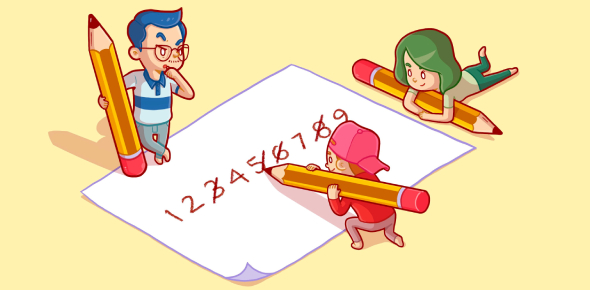# 3rd Grade Math Quiz: MCQ Exam!

15 Questions | Total Attempts: 455Settings.

• 1.
What is \$2.65 - \$2.36?
• A.

290

• B.

0.16

• C.

0.29

• D.

2.9

• 2.
How many millimeters are in a centimeter?
• A.

10

• B.

100

• C.

1000

• D.

1

• 3.
What is 3/6 + 1/6 written in lowest terms?
• A.

12-Apr

• B.

6-Apr

• C.

Mar-36

• D.

3-Feb

• 4.
How do you write 0.68 as a percent?
• A.

68%

• B.

68

• C.

0.68%

• D.

6.80%

• 5.
If a pentagon is divided into 5 equal parts, what would the fraction name for one part be?
• A.

2-Jan

• B.

3-Jan

• C.

4-Jan

• D.

5-Jan

• 6.
What is 29% in decimal form?
• A.

29

• B.

0.29%

• C.

2.9

• D.

0.29

• 7.
What is 1/3 + 2/3 written in lowest terms?
• A.

1

• B.

6-Mar

• C.

9-Mar

• D.

3-Mar

• 8.
If a pizza has 8 equal slices and you ate 2 slices, what part did you eat?
• A.

8-Feb

• B.

8-Jan

• C.

3-Jan

• D.

2-Jan

• 9.
If we divide a triangle into 3 equal parts, what fraction would name one of the parts?
• A.

1

• B.

3-Jan

• C.

3

• D.

4-Jan

• 10.
There are 3 adults and 5 children in the pool, how many people are in the pool?
• A.

5-Mar

• B.

3

• C.

5

• D.

8

• 11.
A sticker costs 75 cents and you pay with a one dollar bill, how much change should you get back?
• A.

1.25

• B.

1

• C.

75 Cents

• D.

25 Cents

• 12.
How do you write 0.862 as a fraction?
• A.

862/10

• B.

862/1000

• C.

862/1

• D.

862/100

• 13.
How many corners does a rectangle have?
• A.

3

• B.

5

• C.

6

• D.

4

• 14.
If you buy a candy bar for 85 cents, and pay with a \$5 bill, how much change should you get back?
• A.

4.85

• B.

0.15

• C.

4.15

• D.

4

• 15.
How do you write 3.6 as a mixed number?
• A.

36/100

• B.

36/10

• C.

3 3/5

• D.

3 3/5

Related TopicsBack to top